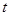# Kinematics in One Dimensions practice problems

In this page we have Kinematics in One Dimensions practice problems . Hope you like them and do not forget to like , social share and comment at the end of the page.

Question 1
(a) Under what condition will the Distance and displacement of a moving object will have same magnitude?
(b) Can a body have a constant velocity but a varying speed?
(c) Can a x-t graph be a straight line parallel to time axis?
(d) Can a x-t graph be a straight line parallel to position axis?
Question 2 Show that area under the velocity time graph of a particle in uniform motion gives the displacement of the particle in a given time.
Question 3 Derive following relations for uniformly accelerated motion using calculus method
(a) Velocity time relation
(b) Position time relation
(c) Velocity displacement relation
Question 4 A car starting from rest, accelerates at a rate $f$ through a distance $S$ , then continues at constant speed for time t  and then decelerates at the rate $\frac{f}{2}$to come to rest. If the total distance traversed is 5S, then prove that $S=\frac{1}{2}ft^{2}$
Question 5 A car starts from rest and accelerates uniformly for 10 s to a velocity 8 m/s. It then runs at a constant velocity and is finally bought to rest in 64 m with a constant retardation. The total distance covered by the car is 584 m. find the value of acceleration, retardation and the total time taken.
Question 6 the relation between time t and distance $x$ is
$t=ax^3+bx$
, where $a$ and $b$ are constants. Find the instantaneous acceleration.

Question 7
(a) Can a body have a constant speed but varying velocity?
(b) A ball is thrown straight up. What is its velocity and acceleration at the top?
(c) Two balls of different masses (one lighter and heavier) are thrown vertically upwards with same initial speed. Which one will rise to the greater height?
(d) Can a body have zero velocity and finite acceleration? Justify your answer with an example.
Question 8 The v-t graph of two objects make angle of 300 and 60 0 with the time axis. Find the ratio of their acceleration.
Question 9 ‘The direction in which the object moves is given by the direction of velocity of the object and not by the direction of acceleration’. Explain the above statement with a suitable example.
Question 10 If the distance covered by a moving object varies directly as the time, what conclusion could you draw about the motion and the forces?
Question 11 An object is covering distance in direct proportion to$t^3$, whereis the time elapsed.
(a) What conclusion might you raw about the acceleration? Is it constant? Increasing? Decreasing? Zero?
(b) What might you conclude about the force acting on the object?